So, how much energy would it take to disintegrate the Earth? Marvin the Martian wants to know!

Let's assume that to completely disintegrate something, we have to move all of its pieces completely away from each other. In our case, we'll consider only the gravitationally bound atoms and molecules that constitute the Earth. The atoms within molecules are bound to one another by the electromagnetic force. We want to stick solely to the force of gravity for this analysis, so we'll not consider the ripping apart of molecules.

By symmetry, the energy required to separate all the Earth's bits is equal in magnitude but opposite in sign to the potential energy lost by the Earth's mass as it assembled itself from the protoplanetary disk that once stood where the solar system now exists. The gravitational binding energy (also called gravitational self energy) of a system of particles is the change in the system's potential energy as all its bits fell to the bottom of the system's gravitational potential well.

Or, we can approach it from the other direction: the binding energy is the opposite of the system's change in potential energy as its bits are moved an infinite distance apart.  This works as well for gravitationally bound systems like planets as it does for molecules bound by the electromagnetic force and atomic nuclei bound by the strong nuclear force. In the case of the  Earth, its gravitational binding energy is equal in magnitude and opposite in sign to the energy required to move its gravitationally bound atoms and molecules an infinite distance apart.

So how do we know how much energy it would take to move all those bits apart? It's simply the change in potential energy as the bits are separated. So how do we find the change in potential energy?  Like this.

Through the work-energy theorem, we know a system's change in potential energy is the negative of the integral of force over a distance. This is discussed in greater depth in the Gravitational Potential essay. For the force of gravity, we havewhere

 ΔUg = Change in gravitational potential energy, J G = 6.6743×10-11 m3·kg-1·s-2 Universal gravitational constant 1 M = Mass of one object, usually the larger, kg m = Mass of another object, usually the smaller, kg r = Distance between the objects' centers of mass, m

To apply this relationship to the problem of finding the gravitational binding energy of the Earth, we imagine removing one infinitesimally thick outer shell after the other from a spherical distribution of mass that gets smaller and smaller as shells are removed. Each mass shell is moved an infinite distance away through the diminishing force of gravity between the shell and sphere. It will take some energy to move all these shells; that energy is the negative of the binding energy of the system. To find energy needed to remove all these shells, we will find the change in the system's potential energy. To find the change in potential energy, we need to know the mass of the shell, the mass of the remainder of the sphere, and the force through which we're moving the shell.

The mass of any volume is simply density times volume. Each shell has a volume given by its area times its thickness and a mass given by its volume times its density. In short, we have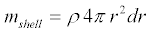where ρ is the density of the shell, r is the radius of the shell, and dr is the thickness of the shell.

The remainder of the sphere inside the shell will have a mass given by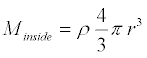where ρ is the density of the sphere and r is the radius of the sphere.

If we assume that the density of the sphere is the same everywhere, then each shell contributes an infinitesimal portion of potential energy as described bywhere the average density is given by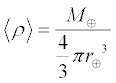where the angle brackets indicate an average value and Å is the astronomical symbol for Earth. (It's actually the Boolean exclusive-or operator, but close enough.)

Substituting, we obtain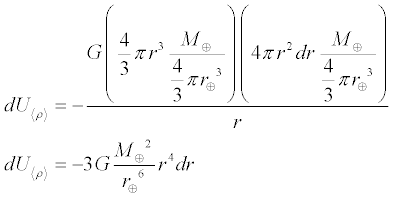To find the total change in potential energy as all the shells are removed by summing all the infinitesimal bits of potential energy, we integrate over the radius of all the shells, from the outside to the inside, like thisThus for any point within the sphere, the change in potential energy using average density isTherefore the total change in potential energy as the Earth is disassembled using average density becomes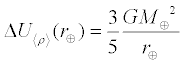If, on the other hand, we do not wish to assume a constant density throughout the Earth, we'll need a density that varies with radius. We can approximate the Earth as a sphere having several layers, each layer having a quadratic density function as described in the Earth Gravity essay. In short, we have a piecewise density function.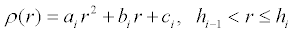where r is the distance from the center of the Earth and whereand where the indexed constants for each piece of the function are described in the table below.

 i Layer Height hi (m) ai (kg·m-5) bi (kg·m-4) ci (kg·m-3) 1 Inner core 1.2215×106 -2.1773×10-10 1.9110×10-8 1.3088×104 2 Outer core 3.4800×106 -2.4123×10-10 1.3976×10-4 1.2346×104 3 D'' layer 3.6300×106 0 -5.0007×10-4 7.3067×103 4 Lower Mantle 5.7010×106 -3.0922×10-11 -2.4441×10-4 6.7823×103 5 Inner transition zone 1 5.7710×106 0 -2.3286×10-4 5.3197×103 6 Inner transition zone 2 5.9710×106 0 -1.2603×10-3 1.1249×104 7 Outer transition zone 6.1510×106 0 -5.9706×10-4 7.1083×103 8 Low velocity zone & lid 6.3466×106 0 1.0869×10-4 2.6910×103 9 Inner crust 6.3560×106 0 0 2.9000×103 10 Outer crust 6.3680×106 0 0 2.6000×103 11 Ocean 6.3710×106 0 0 1.0200×103

Going back to our expression for the mass of the shell and the mass of the sphere inside the shell, we now have the following infinitesimal potential energy, this time using the real density instead of an average density.Same as before, to sum all the infinitesimal bits of binding energy we integrate over the radius of all the shells from the outside in.  This time, however, we can only integrate one layer at a time since the piecewise density function is not differentiable across the layer boundaries.  We'll first derive a relationship for all the infinitesimal shells within one layer, then we'll sum the layers.

For a point having a radius within a given layer, we will need to find the potential energy change that occurs as that layer is stripped, shell by shell, from the radius of the point in question to the inside of the layer. For a point h in a layer having lower and upper bounds of h1 and h2 respectively, we have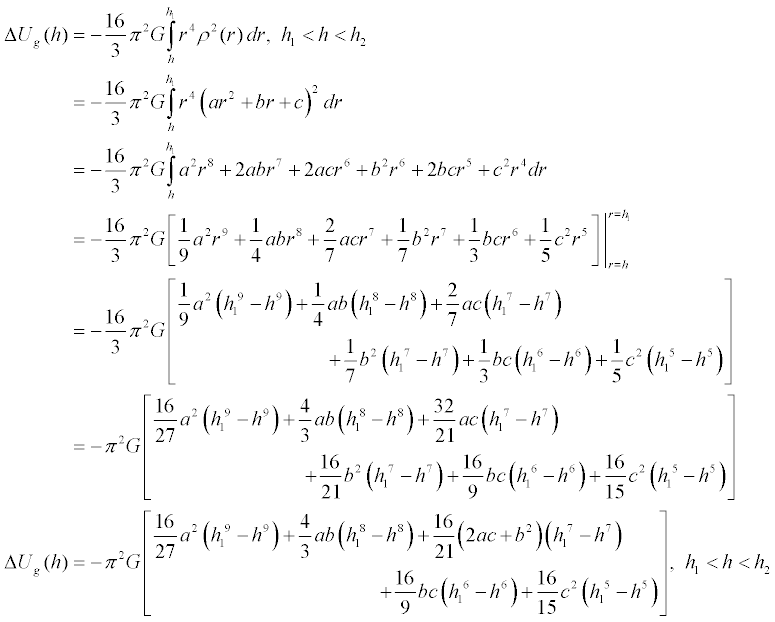Which can be seen to reduce to the equation for average density by assuming a sphere of one layer where a = b = h1 = 0 and c = <ρ>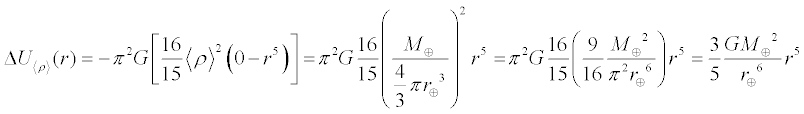We can also see that for the entire ith layer we have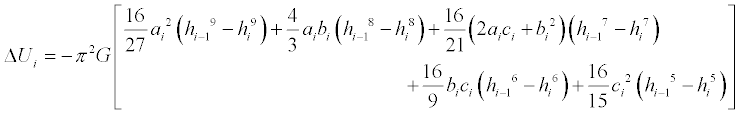and thus for any point r along the radius of the sphere, we have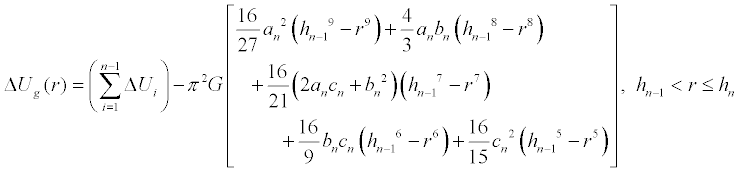where ΔU0 = 0 and the indexed constants for each piece of the function are described in the table above.

And since the gravitational binding energy is the same magnitude but opposite in sign to the change in potential energy as the system is disassembled, we have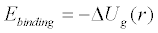Using the average density of the Earth = 5.51394×103 kg·m-3 as calculated in the Earth Gravity essay along with the variable density function discussed above, we obtain the following energies.

Average Density Method:
the Earth's gravitational binding energy is -2.242×1032 J

Variable Density Method:
the Earth's gravitational binding energy is -1.711×1032 J

Thus, while the average density method figure is in the ballpark, it is too high by 31.05%.

Note that the binding energies listed above are negative. This is often a source of unnecessary confusion.

Remember, we define the binding energy as the change in the potential energy of the system as it gravitationally assembled itself. That means the binding energy is also the negative of the change in potential energy as its pieces are separated back to infinity. As the bits are separated, the change in potential energy is positive: it takes an input of energy to separate two masses that are held together by the force of gravity. Therefore, the change in potential energy is negative as the system gravitationally collapsesand therefore the binding energy is negative.

We can also look at this the other way around. If the system requires an energy input to become separated, then the system must have an energy output as it collapses.  It does: in the case of a planet, this energy is called the heat of accretion. All that gravitational potential energy of the protoplanetary disk got converted to heat as all its bits condensed under the force of gravity to form the Sun and the planets. Some of the original gravitational potential energy is still around: the planets have not collapsed into the Sun.

If we plot the average and variable density binding energy functions over the radius of the Earth, we obtain the following; the blue line represents the variable density function and the dashed line is the average density approximation.

Click on the plot thumbnail for a high resolution image best viewed with a 1280x1024 screen resolution.   The Mathematica source code may be found here.

One place in which gravitational binding energy becomes important is in the Nordtvedt effect, which is a test of the equivalence principle envisioned by Kenneth Nordtvedt. 4,5,6,10

The equivalence principle posits that inertial mass—mass used by F = ma, Newton's second law—is identical to gravitational mass—mass used by the law of universal gravitation. Nordtvedt showed that some theories of gravitation predict that gravitational binding energy will affect gravitational mass, but not inertial mass. 6,10

In short, a system of particles loses gravitational potential energy as it undergoes gravitational collapse. As such, the collapsed state has a lower total energy, the difference in energy being the gravitational binding energy.  Since the system has a lower total energy, it also has a lower total mass by E = mc2. Or does it?  The existence of the Nordtvedt effect would mean that gravitational binding energy reduces the gravitational mass of the system as it collapses, while the inertial mass of the system remains constant. 4  As such, perhaps the Earth's gravitational mass is not equal to its inertial mass by a small fraction. But how small a fraction?

We can convert the Earth's binding energies to mass via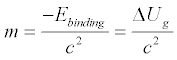where c = the speed of light = 2.99792458×108 m·s-1. 2

Then, we can then find the binding energy mass fraction based on an Earth mass of 5.9736×1024 kg. 3

We end up with the following figures for the Earth.

 Method Binding Energy (J) Mass Equivalent (kg) Mass Fraction Earth Average Density -2.242×1032 2.495×1015 4.177×10-10 Earth Variable Density -1.711×1032 1.904×1015 3.187×10-10

Thus, the Earth's gravitational mass might be less that its inertial mass by up to 320 parts per trillion. So, how might we go about detecting this difference? When Nordtvedt discussed the effect, he came up with the idea of using the Moon as a reference. We can perform the same calculations for the Moon and obtain

 Method Binding Energy (J) Mass Equivalent (kg) Mass Fraction Moon Average Density -1.245×1029 1.385×1012 1.885×10-11 Moon Dual Density -1.223×1029 1.361×1012 1.852×10-11
where 3,7
 Lunar mass = 7.349×1022 kg Lunar volumetric radius = 1.7371×106 m Average lunar density = 3.347×103 kg·m-3 Lunar core radius = 3.50×105 m Lunar core constant density = 7.2 kg·m-3 Lunar mantle constant density = 3.315×103 kg·m-3

In this case, the average density method figure is only 1.80% above the dual density figure. This is expected; the core is dense but very small; and, we're assuming a constant density in the core and the mantle. Having a better density profile for the Moon would make the variable density function more accurate.

If we plot the average and variable density binding energy functions over the radius of the Moon, we obtain the following; the blue line represents the dual density function and the dashed line is the average density approximation.

Click on the plot thumbnail for a high resolution image best viewed with a 1280x1024 screen resolution.
The Mathematica source code may be found here.

Even though the lunar density profile isn't very accurate, it's accurate enough to show that the gravitational binding energy mass fraction of the Moon—20 parts per trillion—is over an order of magnitude smaller than that of the Earth.  That being the case, if the Nordtvedt effect exists, then the Moon would react to the Sun's gravitational field to a greater degree than the Earth. The Moon's ratio of gravitational mass to inertial mass would be higher than the Earth's and the equivalence principle would be shown to have a loophole. If the Nordtvedt effect operates at its theoretical maximum, the Moon's orbit should be elongated along the Earth-Sun radial by an amount on the order of ten meters. 4

Using the retroreflectors left on the lunar surface during the Apollo missions, the Moon's distance can be determined with lasers to millimeter accuracy. 8  To date, the Moon's orbit has not been observed to be measurably polarized along the Earth-Sun radial to any degree at all. The Nordtvedt effect remains unobserved. 8,9,10,11,12  Therefore, if the Nordtvedt effect exists at all, it is quite small.

Notwithstanding possible violations of the equivalence principle, Marvin has his work cut out for him.

The power output of the Sun is 3.846×1026 Watts.2  The complete gravitational disintegration of the Earth would require an input of energy equivalent to the Sun's total output for a continuous 5 days, 3 hours and 34 minutes. Perhaps ten days with a hyperbolic mirror of fractional AU aperture. Place the Sun at one focus and the Earth at the other. It won't be cheap.

One megatonne TNT nuclear equivalent is 4.184×1015 Joules, so you'd need about 1.4 million-billion 30 megatonne thermonuclear warheads to blow up the planet.13  Not even in General Curtis LeMay's wildest dreams would such an arsenal exist, although the Earth certainly contains enough fissionable and fusible material.

Antimatter has the advantage of total annihilation with matter, converting 200% of its mass energy to pure energy in so doing. As such, one would require 950 billion metric tons of antimatter to do the job. One will also need a large containment vessel and a nearly infinite supply of patience as Fermilab's current antiproton production capacity is about 1.5 nanograms per year. 14